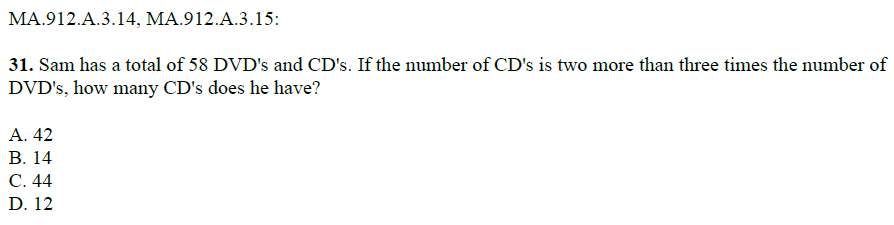# Question 31: Sam, CD's, and DVD's

Algebra Level 2MA.912.A.3.14 - Solve systems of linear equations and inequalities in two and three variables using graphical, substitution, and elimination methods.

MA.912.A.3.15 - Solve real-world problems involving systems of linear equations and inequalities in two and three variables.

Algebra 1 EOC Study Guide. How many can you solve correctly?

×